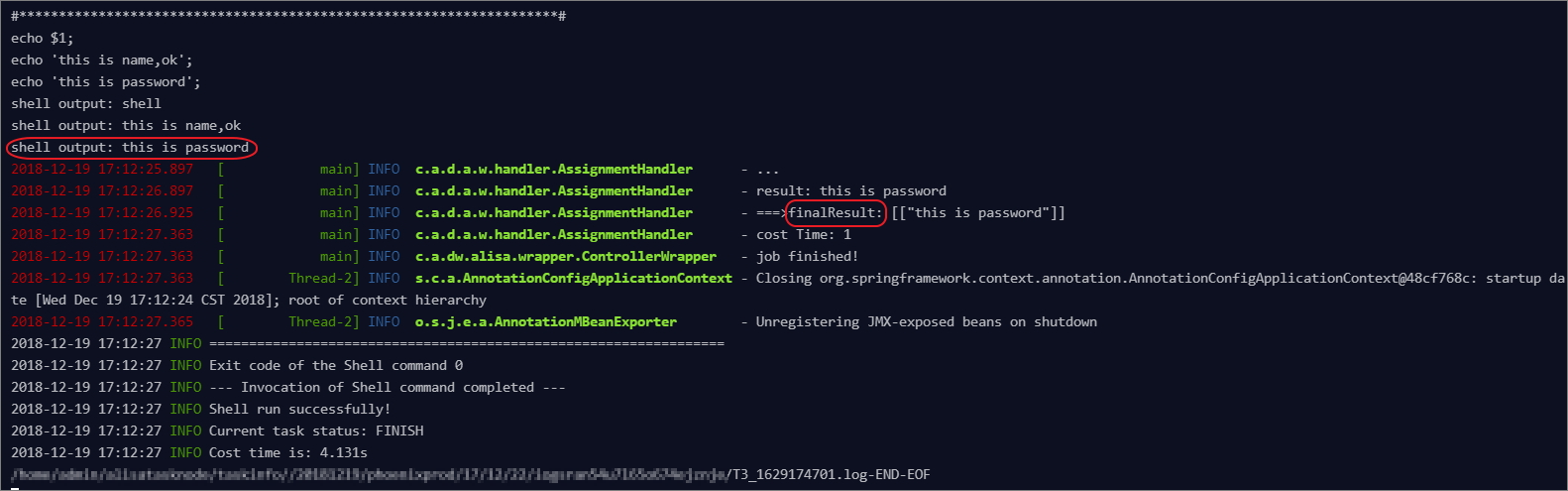This topic describes the functions of the Assignment Node. The Assignment Node is a special node type that supports the assignment of output parameters by writing code in the node. The Assignment Node transfers the integrated node context to downstream nodes for reference, which in turn is used as values.

## Create an assignment node

Go to Control and click the Assignment Node that is located in the class directory of the new node menu, as shown in the following figure.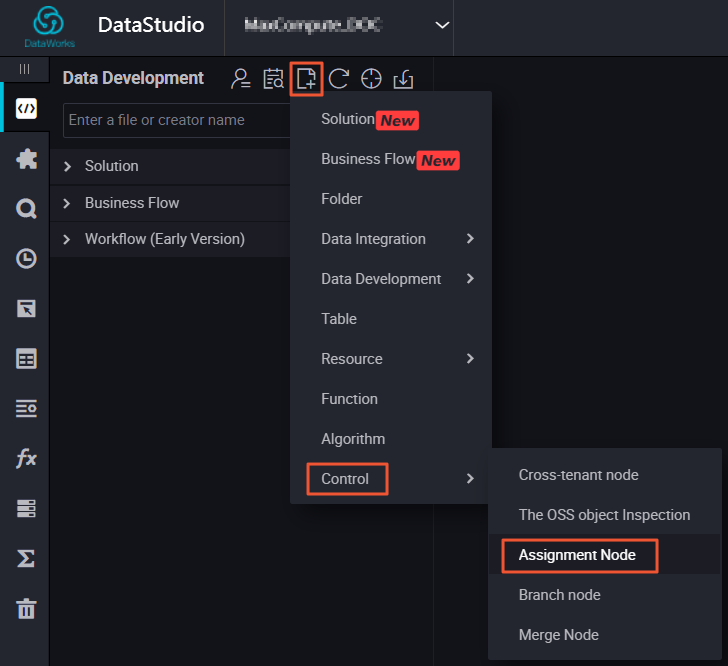## Write the logic value of the assignment node

The assignment node has a fixed output parameter that names outputs in the Node Context. It supports the usage of MaxCompute, Shell, and Python to write code to assign parameters, whose values are the operation and calculation results of the node code. Only one language can be selected for a single assignment node.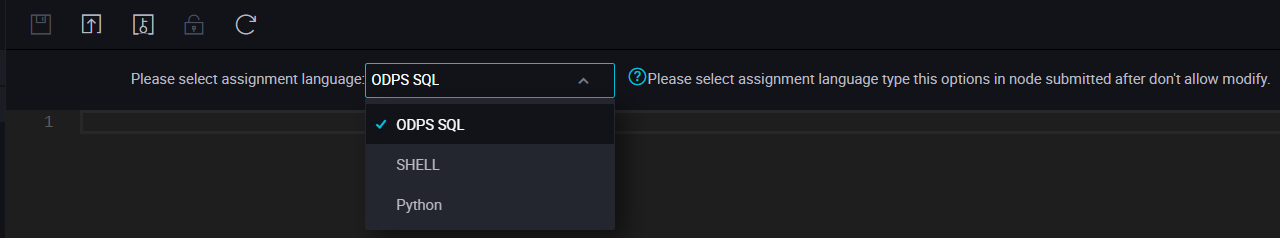Note
• The value of the output parameter takes only the output from the last line of code as follows:
• The output of the SELECT statement on the last line of MaxCompute SQL.
• The data from the ECHO statement on the last line of shell.
• The output of the PRINT statement on the last line of Python.
• The maximum transfer value of the output parameter is 2M. If the assignment statement output value exceeds this limit, the assignment node will fail to run.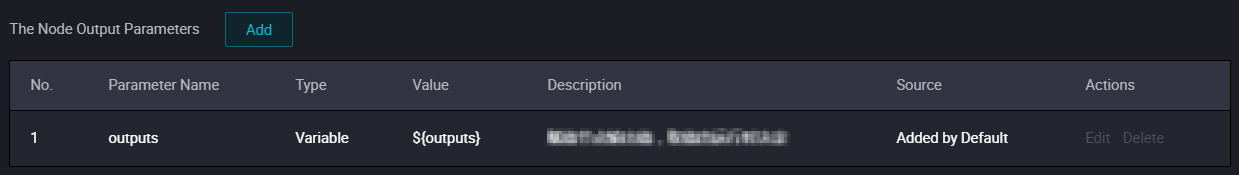## Use the assignment node output on the downstream node

Add an Assignment Node as an upstream dependency in the downstream node, and define the Assignment Node output as an input parameter for the node through node context. Then reference the node in code to obtain the specific values of the upstream assignment node output parameters. For more information, seeNode context.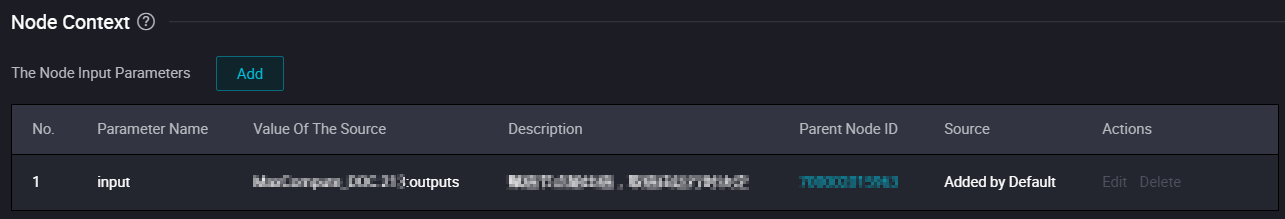## An example of assignment node

1. Create the business flow, and then create the following nodes as shown in the figure, respectively.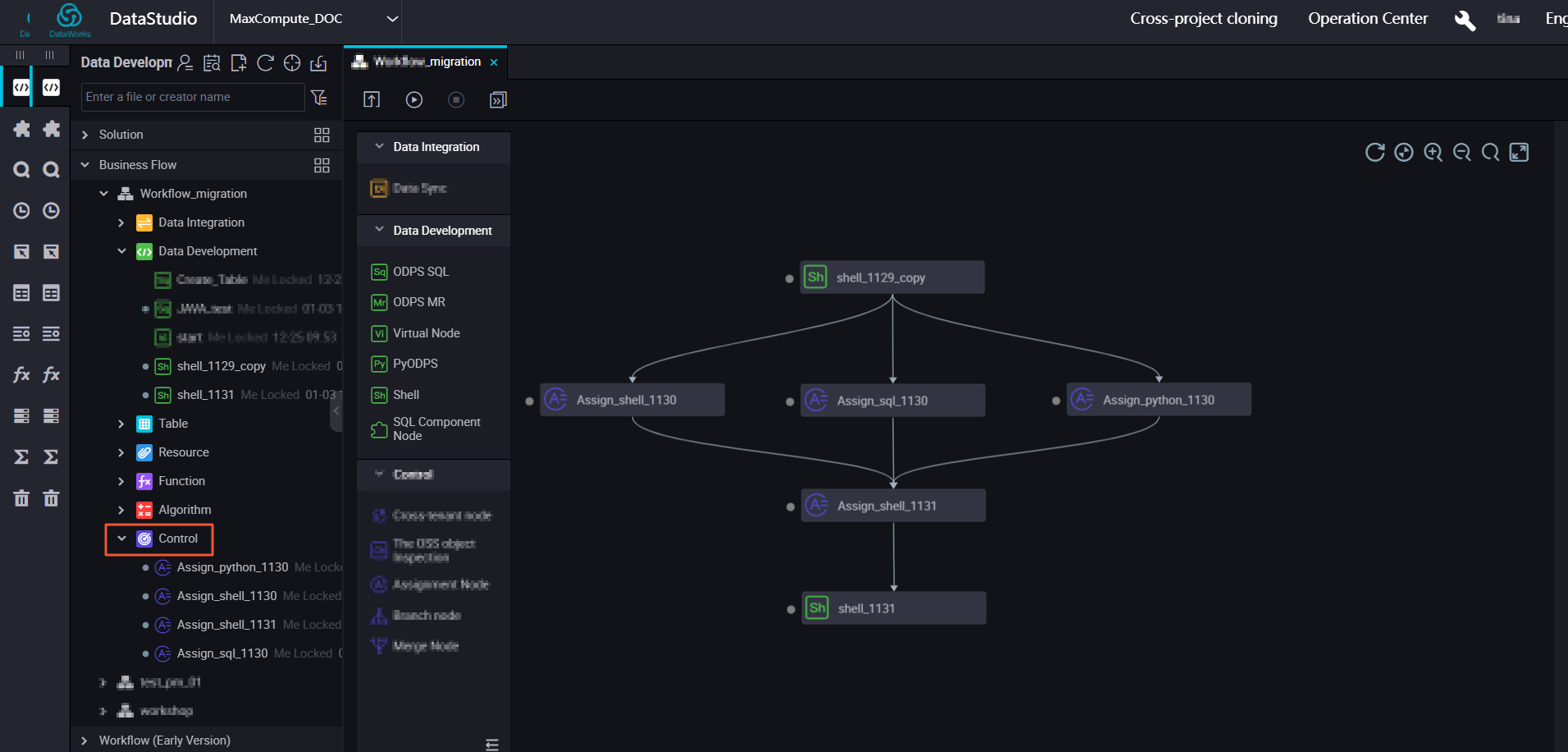2. By default, the system will display an Outputs parameter when the assignment node is configured. After the task is run, you can find the relevant parameter results in the related Operation Center > Properties > Context page.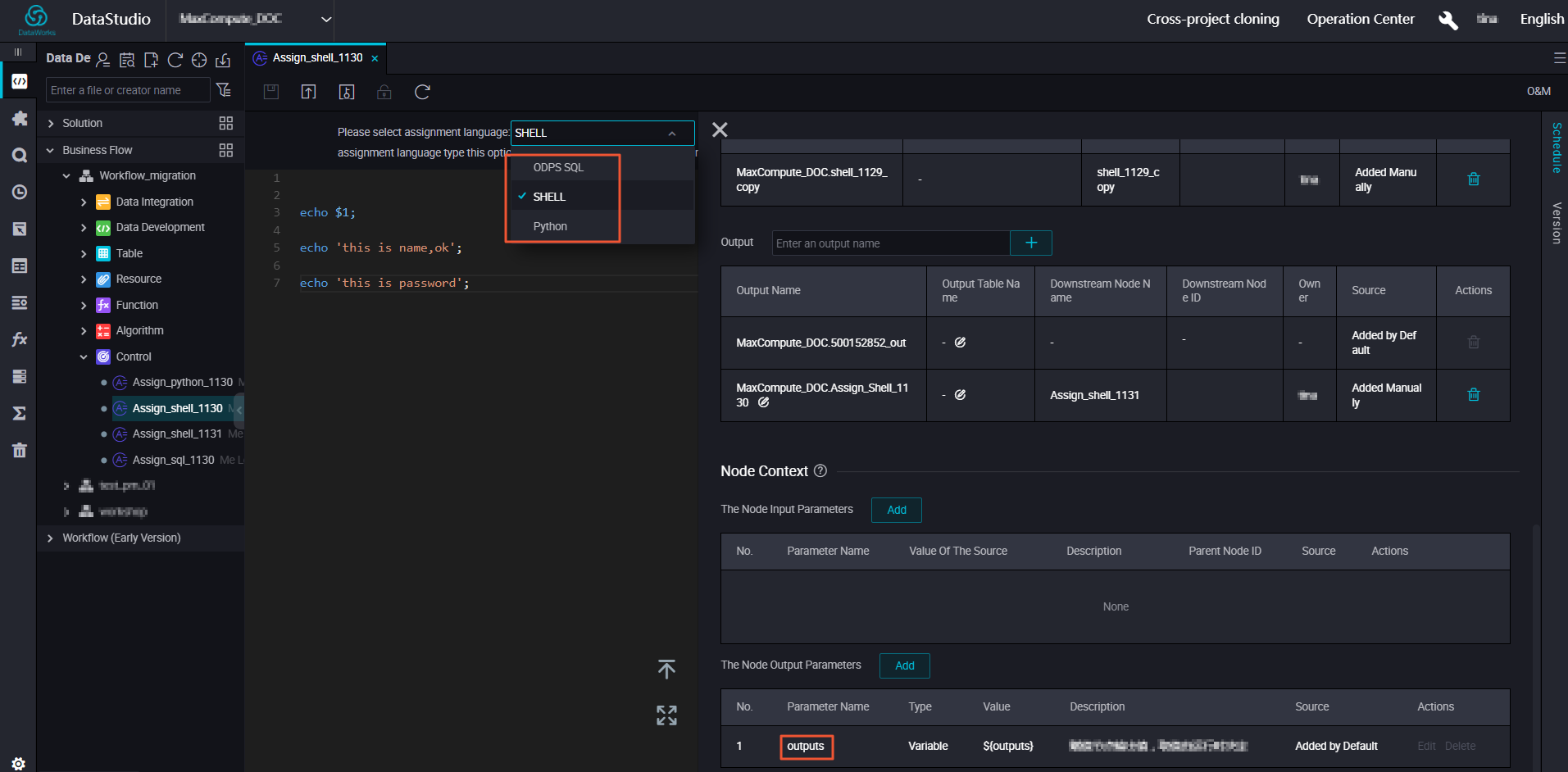3. The upstream Outputs parameter is used as the downstream input parameter, as shown in the figure below.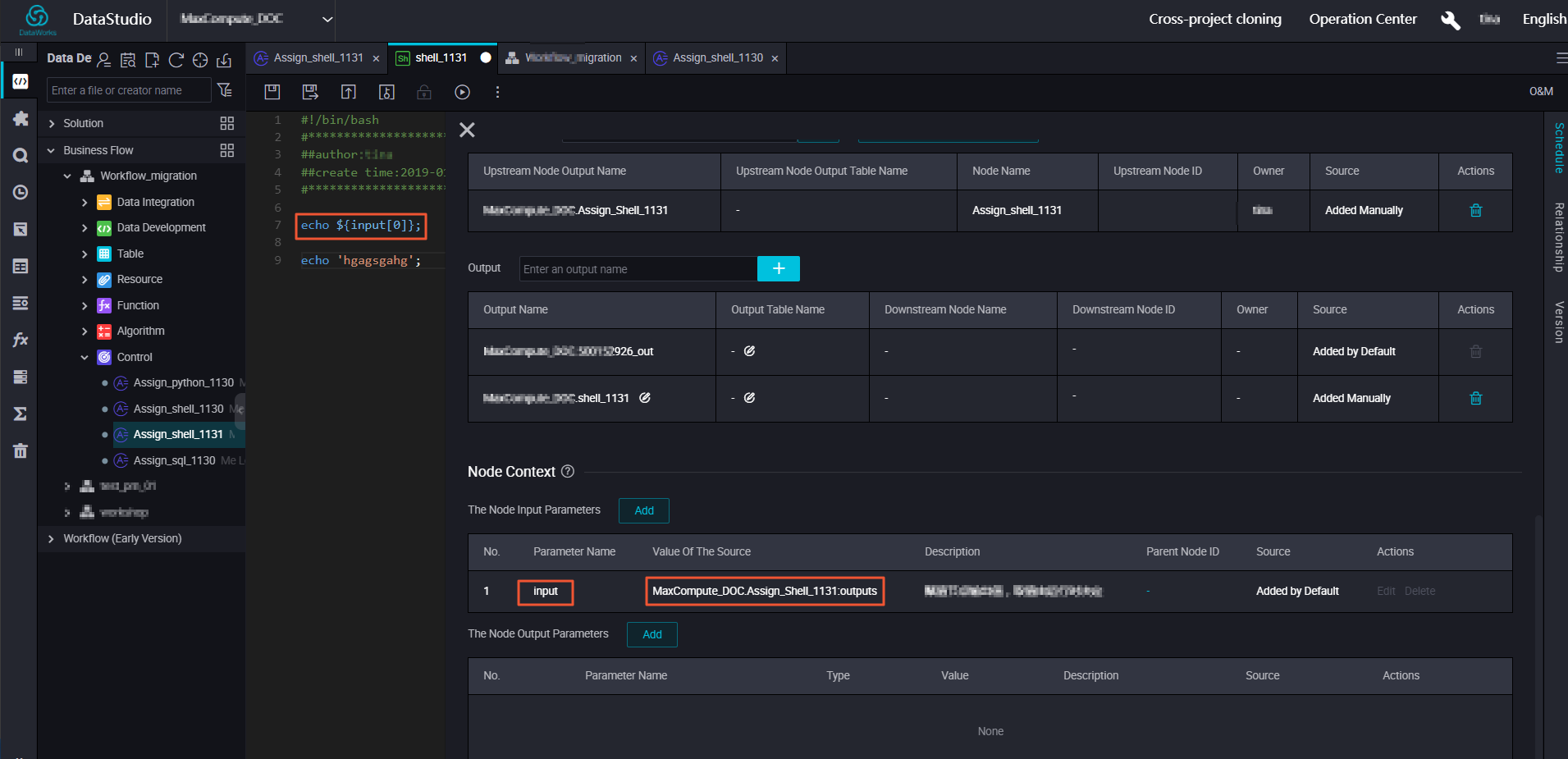## Run the assignment node task

Note Typically, you can supplement data running in the above configuration parameters in O&M. The above configuration parameters can be validated through patch data operation, but the test operation parameters cannot be validated.
1. When the task is configured and scheduled, a run instance is generally generated the next day. The following figure is an example of running supplementary data.
2. You can view the context input and output parameters, and click the next link to view the input or output results during runtime.
3. In the Running Log, you can view the final code output through 'finalResult'.